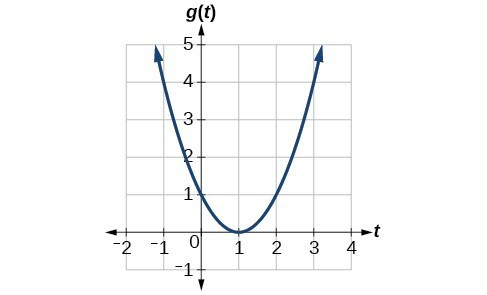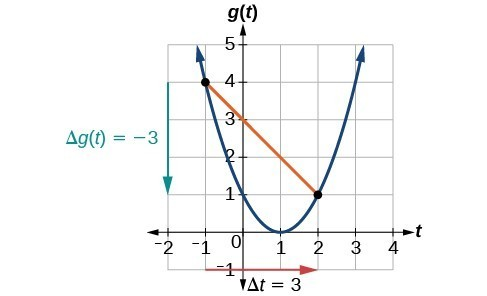## Find the average rate of change of a function

 $y$ 2005 2006 2007 2008 2009 2010 2011 2012 $C\left(y\right)$ 2.31 2.62 2.84 3.3 2.41 2.84 3.58 3.68

The price change per year is a rate of change because it describes how an output quantity changes relative to the change in the input quantity. We can see that the price of gasoline in the table above did not change by the same amount each year, so the rate of change was not constant. If we use only the beginning and ending data, we would be finding the average rate of change over the specified period of time. To find the average rate of change, we divide the change in the output value by the change in the input value.

Average rate of change=$\frac{\text{Change in output}}{\text{Change in input}}$
=$\frac{\Delta y}{\Delta x}$
=$\frac{{y}_{2}-{y}_{1}}{{x}_{2}-{x}_{1}}$
=$\frac{f\left({x}_{2}\right)-f\left({x}_{1}\right)}{{x}_{2}-{x}_{1}}$

The Greek letter $\Delta$ (delta) signifies the change in a quantity; we read the ratio as “delta-y over delta-x” or “the change in $y$ divided by the change in $x$.” Occasionally we write $\Delta f$ instead of $\Delta y$, which still represents the change in the function’s output value resulting from a change to its input value. It does not mean we are changing the function into some other function.

In our example, the gasoline price increased by $1.37 from 2005 to 2012. Over 7 years, the average rate of change was $\frac{\Delta y}{\Delta x}=\frac{{1.37}}{\text{7 years}}\approx 0.196\text{ dollars per year}$ On average, the price of gas increased by about 19.6¢ each year. Other examples of rates of change include: • A population of rats increasing by 40 rats per week • A car traveling 68 miles per hour (distance traveled changes by 68 miles each hour as time passes) • A car driving 27 miles per gallon (distance traveled changes by 27 miles for each gallon) • The current through an electrical circuit increasing by 0.125 amperes for every volt of increased voltage • The amount of money in a college account decreasing by$4,000 per quarter

### A General Note: Rate of Change

A rate of change describes how an output quantity changes relative to the change in the input quantity. The units on a rate of change are “output units per input units.”

The average rate of change between two input values is the total change of the function values (output values) divided by the change in the input values.

$\frac{\Delta y}{\Delta x}=\frac{f\left({x}_{2}\right)-f\left({x}_{1}\right)}{{x}_{2}-{x}_{1}}$

### How To: Given the value of a function at different points, calculate the average rate of change of a function for the interval between two values ${x}_{1}$ and ${x}_{2}$.

1. Calculate the difference ${y}_{2}-{y}_{1}=\Delta y$.
2. Calculate the difference ${x}_{2}-{x}_{1}=\Delta x$.
3. Find the ratio $\frac{\Delta y}{\Delta x}$.

### Example 1: Computing an Average Rate of Change

Using the data in the table below, find the average rate of change of the price of gasoline between 2007 and 2009.

 $y$ 2005 2006 2007 2008 2009 2010 2011 2012 $C\left(y\right)$ 2.31 2.62 2.84 3.3 2.41 2.84 3.58 3.68

### Solution

In 2007, the price of gasoline was $2.84. In 2009, the cost was$2.41. The average rate of change is

$\begin{cases}\frac{\Delta y}{\Delta x}=\frac{{y}_{2}-{y}_{1}}{{x}_{2}-{x}_{1}}\\ {}\\=\frac{2.41-2.84}{2009 - 2007}\\ {}\\=\frac{-0.43}{2\text{ years}}\\{} \\={-0.22}\text{ per year}\end{cases}$

### Analysis of the Solution

Note that a decrease is expressed by a negative change or “negative increase.” A rate of change is negative when the output decreases as the input increases or when the output increases as the input decreases.

The following video provides another example of how to find the average rate of change between two points from a table of values.

### Try It 1

Using the data in the table below, find the average rate of change between 2005 and 2010.

 $y$ 2005 2006 2007 2008 2009 2010 2011 2012 $C\left(y\right)$ 2.31 2.62 2.84 3.3 2.41 2.84 3.58 3.68

Solution

### Example 2: Computing Average Rate of Change from a Graph

Given the function $g\left(t\right)$ shown in Figure 1, find the average rate of change on the interval $\left[-1,2\right]$.Figure 1

### SolutionFigure 2

At $t=-1$, the graph shows $g\left(-1\right)=4$. At $t=2$, the graph shows $g\left(2\right)=1$.

The horizontal change $\Delta t=3$ is shown by the red arrow, and the vertical change $\Delta g\left(t\right)=-3$ is shown by the turquoise arrow. The output changes by –3 while the input changes by 3, giving an average rate of change of

$\frac{1 - 4}{2-\left(-1\right)}=\frac{-3}{3}=-1$

### Analysis of the Solution

Note that the order we choose is very important. If, for example, we use $\frac{{y}_{2}-{y}_{1}}{{x}_{1}-{x}_{2}}$, we will not get the correct answer. Decide which point will be 1 and which point will be 2, and keep the coordinates fixed as $\left({x}_{1},{y}_{1}\right)$ and $\left({x}_{2},{y}_{2}\right)$.

### Example 3: Computing Average Rate of Change from a Table

After picking up a friend who lives 10 miles away, Anna records her distance from home over time. The values are shown in the table below. Find her average speed over the first 6 hours.

 t (hours) 0 1 2 3 4 5 6 7 D(t) (miles) 10 55 90 153 214 240 282 300

### Solution

Here, the average speed is the average rate of change. She traveled 282 miles in 6 hours, for an average speed of

$\begin{cases}\\ \frac{292 - 10}{6 - 0}\\ {}\\ =\frac{282}{6}\\{}\\ =47 \end{cases}$

The average speed is 47 miles per hour.

### Analysis of the Solution

Because the speed is not constant, the average speed depends on the interval chosen. For the interval [2,3], the average speed is 63 miles per hour.

### Example 4: Computing Average Rate of Change for a Function Expressed as a Formula

Compute the average rate of change of $f\left(x\right)={x}^{2}-\frac{1}{x}$ on the interval $\text{[2,}\text{4].}$

### Solution

We can start by computing the function values at each endpoint of the interval.

$\begin{cases}f\left(2\right)={2}^{2}-\frac{1}{2}& f\left(4\right)={4}^{2}-\frac{1}{4} \\ =4-\frac{1}{2} & =16-{1}{4} \\ =\frac{7}{2} & =\frac{63}{4} \end{cases}$

Now we compute the average rate of change.

$\begin{cases}\text{Average rate of change}=\frac{f\left(4\right)-f\left(2\right)}{4 - 2}\hfill \\{}\\\text{ }=\frac{\frac{63}{4}-\frac{7}{2}}{4 - 2}\hfill \\{}\\� \text{ }\text{ }=\frac{\frac{49}{4}}{2}\hfill \\ {}\\ \text{ }=\frac{49}{8}\hfill \end{cases}$

The following video provides another example of finding the average rate of change of a function given a formula and an interval.

### Try It 2

Find the average rate of change of $f\left(x\right)=x - 2\sqrt{x}$ on the interval $\left[1,9\right]$.

Solution

### Example 5: Finding the Average Rate of Change of a Force

The electrostatic force $F$, measured in newtons, between two charged particles can be related to the distance between the particles $d$, in centimeters, by the formula $F\left(d\right)=\frac{2}{{d}^{2}}$. Find the average rate of change of force if the distance between the particles is increased from 2 cm to 6 cm.

### Solution

We are computing the average rate of change of $F\left(d\right)=\frac{2}{{d}^{2}}$ on the interval $\left[2,6\right]$.

$\begin{cases}\text{Average rate of change }=\frac{F\left(6\right)-F\left(2\right)}{6 - 2}\\ {}\\ =\frac{\frac{2}{{6}^{2}}-\frac{2}{{2}^{2}}}{6 - 2} & \text{Simplify}. \\ {}\\=\frac{\frac{2}{36}-\frac{2}{4}}{4}\\{}\\ =\frac{-\frac{16}{36}}{4}\text{Combine numerator terms}.\\ {}\\=-\frac{1}{9}\text{Simplify}\end{cases}$

The average rate of change is $-\frac{1}{9}$ newton per centimeter.

### Example 6: Finding an Average Rate of Change as an Expression

Find the average rate of change of $g\left(t\right)={t}^{2}+3t+1$ on the interval $\left[0,a\right]$. The answer will be an expression involving $a$.

### Solution

We use the average rate of change formula.

$\text{Average rate of change}=\frac{g\left(a\right)-g\left(0\right)}{a - 0}\text{Evaluate}$.
=$\frac{\left({a}^{2}+3a+1\right)-\left({0}^{2}+3\left(0\right)+1\right)}{a - 0}\text{Simplify}.$
=$\frac{{a}^{2}+3a+1 - 1}{a}\text{Simplify and factor}.$
=$\frac{a\left(a+3\right)}{a}\text{Divide by the common factor }a.$
=$a+3$

This result tells us the average rate of change in terms of $a$ between $t=0$ and any other point $t=a$. For example, on the interval $\left[0,5\right]$, the average rate of change would be $5+3=8$.

### Try It 3

Find the average rate of change of $f\left(x\right)={x}^{2}+2x - 8$ on the interval $\left[5,a\right]$.

Solution